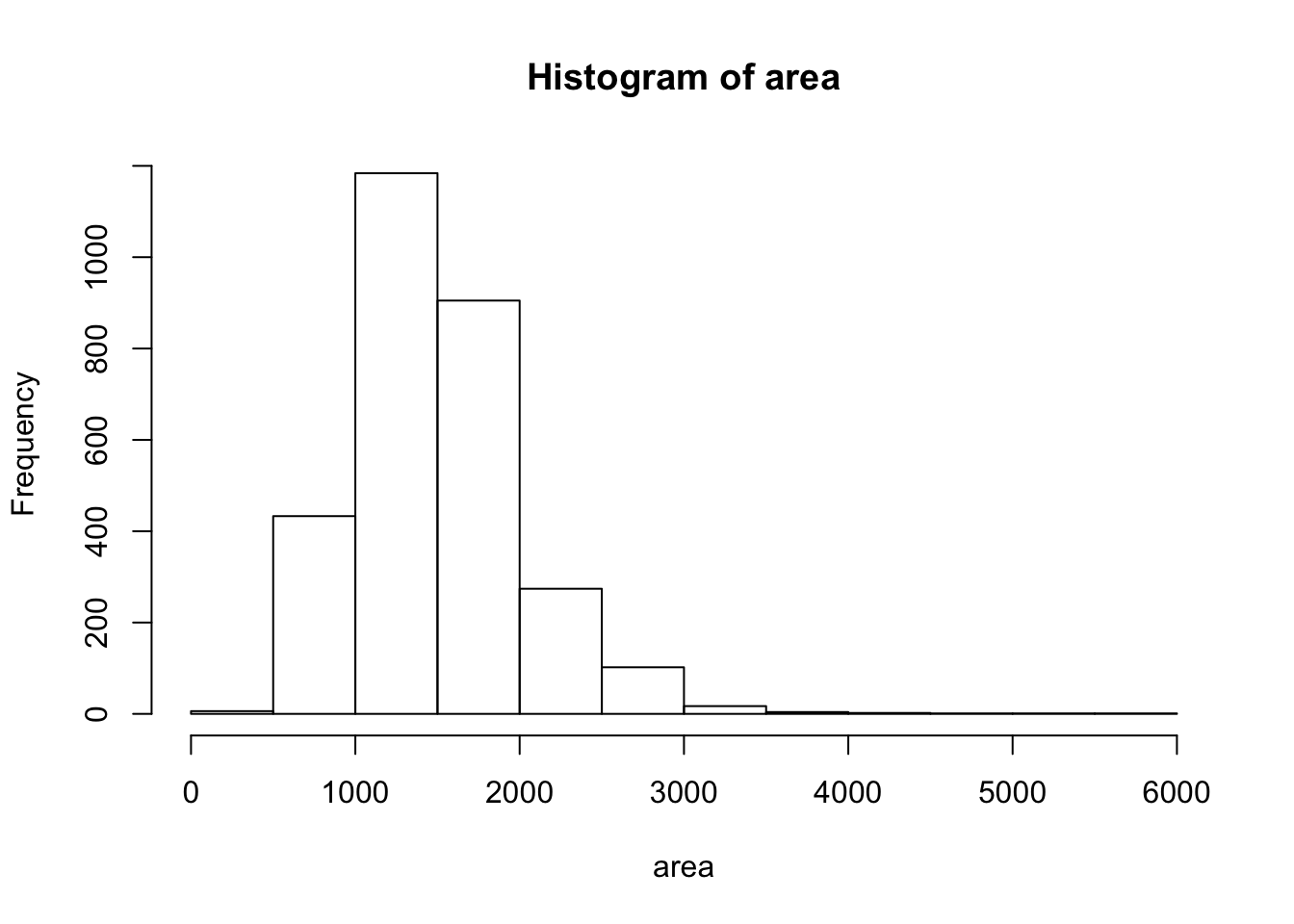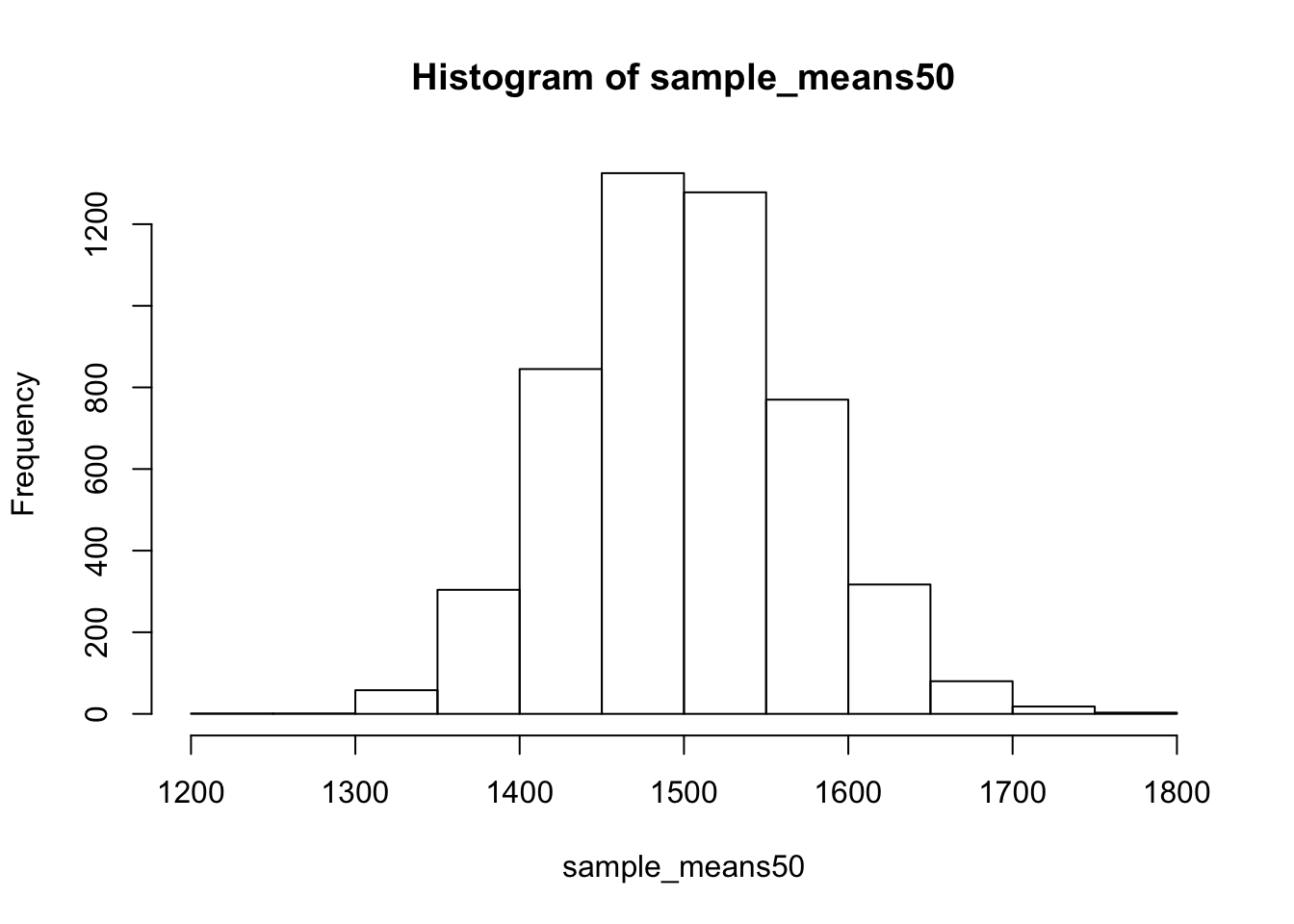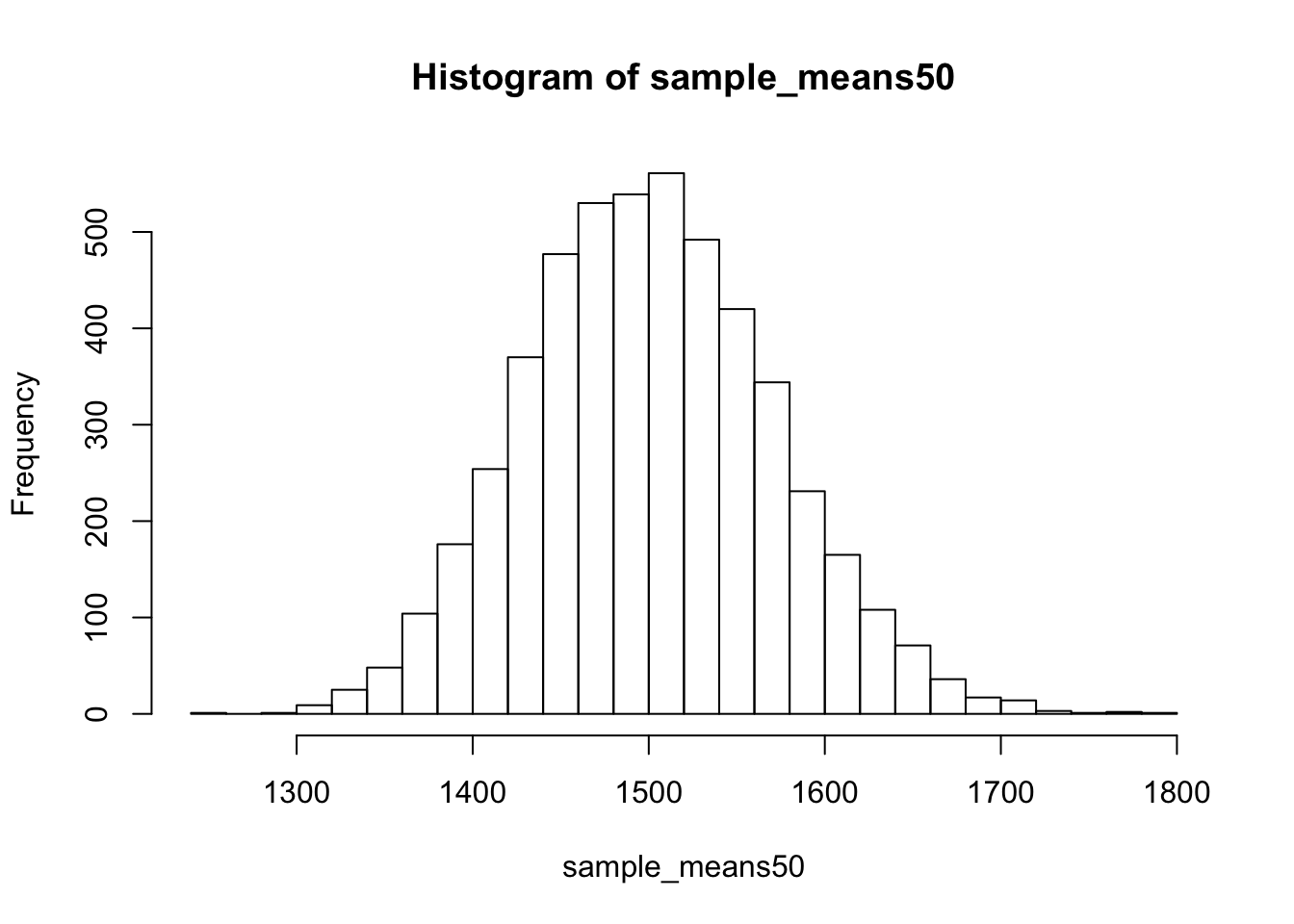This tutorial shows you:

• how to sample from a variable
• how to create a sampling distribution
• how to use for-loops in R
• how to calculate and plot confidence intervals

Note on copying & pasting code from the PDF version of this tutorial: Please note that you may run into trouble if you copy & paste code from the PDF version of this tutorial into your R script. When the PDF is created, some characters (for instance, quotation marks or indentations) are converted into non-text characters that R won’t recognize. To use code from this tutorial, please type it yourself into your R script or you may copy & paste code from the source file for this tutorial which is posted on my website.

# Saving your code (as usual)

Click on File -> New -> R Markdown. This will open a blank document above the console. As you go along you can copy and paste your code into R code chunks and save it. This is a good way to keep track of your code and be able to reuse it later. To run your code from this document, highlight the code and hit the Run button, or highlight the code and hit command+enter or a Mac or control+enter on a PC.

You’ll also want to save your R Markdown file. To do so click on the disk icon. The first time you hit save, RStudio will ask for a file name; you can name it anything you like. Once you hit save you’ll see the file appear under the Files tab in the lower right panel. You can reopen this file anytime by simply clicking on it.

# Background

In the first part of this tutorial, we investigate the ways in which the statistics from a random sample of data can serve as point estimates for population parameters. We’re interested in formulating a sampling distribution of our estimate in order to learn about the properties of the estimate, such as its distribution.

## The data

We consider real estate data from the city of Ames, Iowa. The details of every real estate transaction in Ames is recorded by the City Assessor’s office. Our particular focus for this tutorial will be all residential home sales in Ames between 2006 and 2010. This collection represents our population of interest. In this tutorial we would like to learn about these home sales by taking smaller samples from the full population. Let’s load the data.

``ames <- read.csv("http://www.jkarreth.net/files/ames.csv")``

We see that there are quite a few variables in the data set, enough to do a very in-depth analysis. For this tutorial, we’ll restrict our attention to just two of the variables: the above ground living area of the house in square feet (`Gr.Liv.Area`) and the sale price (`SalePrice`). To save some effort throughout the tutorial, create two variables with short names that represent these two variables.

``````area <- ames\$Gr.Liv.Area
price <- ames\$SalePrice``````

Let’s look at the distribution of area in our population of home sales by calculating a few summary statistics and making a histogram.

``summary(area)``
``````##    Min. 1st Qu.  Median    Mean 3rd Qu.    Max.
##     334    1126    1442    1500    1743    5642``````
``hist(area)``# The unknown sampling distribution

In this tutorial we have access to the entire population, but this is rarely the case in real life. Gathering information on an entire population is often extremely costly or impossible. Because of this, we often take a sample of the population and use that to understand the properties of the population.

If we were interested in estimating the mean living area in Ames based on a sample, we can use the following command to survey the population.

``samp1 <- sample(area, 50)``

This command collects a simple random sample of size 50 from the vector `area`, which is assigned to `samp1`. This is like going into the City Assessor’s database and pulling up the files on 50 random home sales. Working with these 50 files would be considerably simpler than working with all 2930 home sales.

If we’re interested in estimating the average living area in homes in Ames using the sample, our best single guess is the sample mean.

``mean(samp1)``
``##  1604.38``

Depending on which 50 homes you selected, your estimate could be a bit above or a bit below the true population mean of 1499.69 square feet. In general, though, the sample mean turns out to be a pretty good estimate of the average living area, and we were able to get it by sampling less than 3% of the population.

Not surprisingly, every time we take another random sample, we get a different sample mean. It’s useful to get a sense of just how much variability we should expect when estimating the population mean this way. The distribution of sample means, called the sampling distribution, can help us understand this variability. In this tutorial, because we have access to the population, we can build up the sampling distribution for the sample mean by repeating the above steps many times. Here we will generate 5000 samples and compute the sample mean of each.

``````sample_means50 <- rep(NA, 5000)

for(i in 1:5000){
samp <- sample(area, 50)
sample_means50[i] <- mean(samp)
}

hist(sample_means50)``````If you would like to adjust the bin width of your histogram to show a little more detail, you can do so by changing the `breaks` argument.

``hist(sample_means50, breaks = 25)``Here we use R to take 5000 samples of size 50 from the population, calculate the mean of each sample, and store each result in a vector called `sample_means50`. On the next page, we’ll review how this set of code works.

## Interlude: The `for` loop

Let’s take a break from the statistics for a moment to let that last block of code sink in. You have just run your first `for` loop, a cornerstone of computer programming. The idea behind the for loop is iteration: it allows you to execute code as many times as you want without having to type out every iteration. In the case above, we wanted to iterate the two lines of code inside the curly braces that take a random sample of size 50 from `area` then save the mean of that sample into the `sample_means50` vector. Without the `for` loop, this would be painful:

``````sample_means50 <- rep(NA, 5000)

samp <- sample(area, 50)
sample_means50 <- mean(samp)

samp <- sample(area, 50)
sample_means50 <- mean(samp)

samp <- sample(area, 50)
sample_means50 <- mean(samp)

samp <- sample(area, 50)
sample_means50 <- mean(samp)``````

and so on…

With the for loop, these thousands of lines of code are compressed into a handful of lines. We’ve added one extra line to the code below, which prints the variable `i` during each iteration of the `for` loop. Run this code.

``````sample_means50 <- rep(NA, 5000)

for(i in 1:5000){
samp <- sample(area, 50)
sample_means50[i] <- mean(samp)
print(i)
}``````

Let’s consider this code line by line to figure out what it does. In the first line we initialized a vector. In this case, we created a vector of 5000 zeros called `sample_means50`. This vector will will store values generated within the `for` loop.

The second line calls the `for` loop itself. The syntax can be loosely read as, “for every element `i` from 1 to 5000, run the following lines of code”. You can think of `i` as the counter that keeps track of which loop you’re on. Therefore, more precisely, the loop will run once when `i = 1`, then once when `i = 2`, and so on up to `i = 5000`.

The body of the `for` loop is the part inside the curly braces, and this set of code is run for each value of `i`. Here, on every loop, we take a random sample of size 50 from `area`, take its mean, and store it as the \(i\)th element of `sample_means50`.

In order to display that this is really happening, we asked R to print `i` at each iteration. This line of code is optional and is only used for displaying what’s going on while the `for` loop is running.

The `for` loop allows us to not just run the code 5000 times, but to neatly package the results, element by element, into the empty vector that we initialized at the outset.

## Sample size and the sampling distribution

Mechanics aside, let’s return to the reason we used a `for` loop: to compute a sampling distribution, specifically, this one.

``hist(sample_means50)``